Transformer 近两年非常火爆，内容也很多，要想讲清楚，还涉及一些基于该结构的预训练模型，例如著名的 BERT，GPT，以及刚出的 DALLE 等。

Transformer 是 Google 在 2017 年提出的用于机器翻译的模型。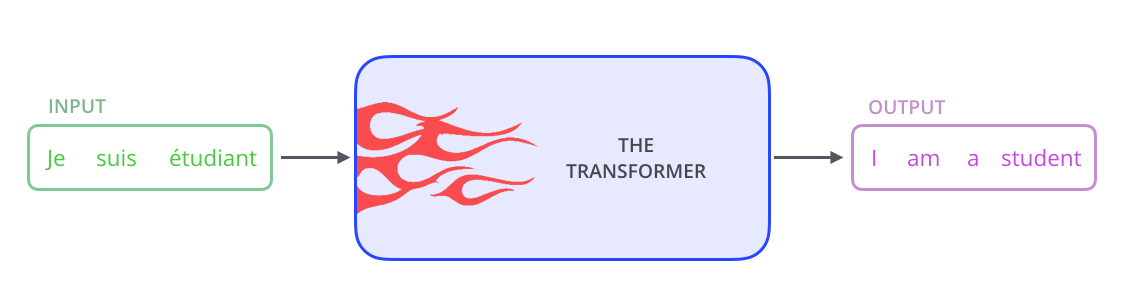Transformer 的内部，在本质上是一个 Encoder-Decoder 的结构，即 编码器-解码器。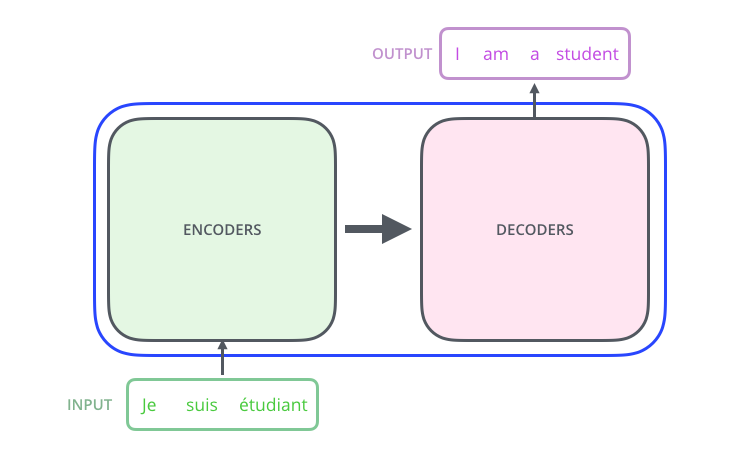Transformer 中抛弃了传统的 CNN和 RNN，整个网络结构完全由 Attention机制组成，并且采用了 6 层 Encoder-Decoder 结构。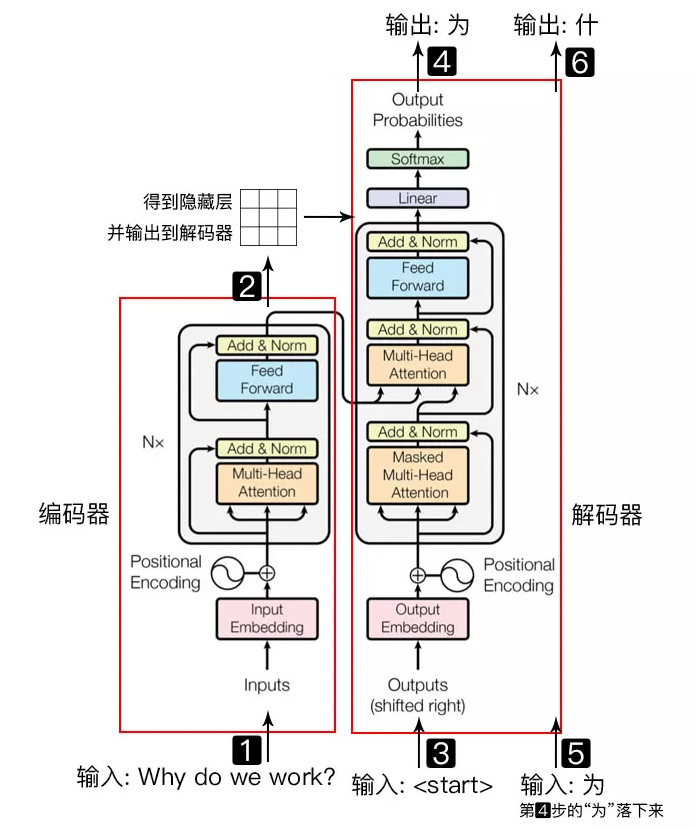• 输入自然语言序列到编码器: Why do we work?(为什么要工作)；
• 编码器输出的隐藏层，再输入到解码器；
• 输入 start （起始）符号到解码器；
• 解码器得到第一个字"为"；
• 将得到的第一个字"为"落下来再输入到解码器；
• 解码器得到第二个字"什"；
• 将得到的第二字再落下来，直到解码器输出 end（终止符），即序列生成完成。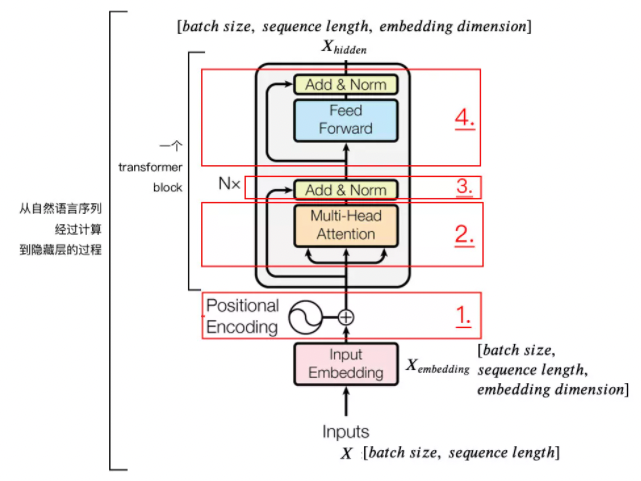## 1. 位置嵌入（positional encoding）

batch size就是 batch的大小，这里只有一句话，所以 batch size为 1，sequence length 是句子的长度，一共 7个字，所以输入的数据维度是 [1, 7]。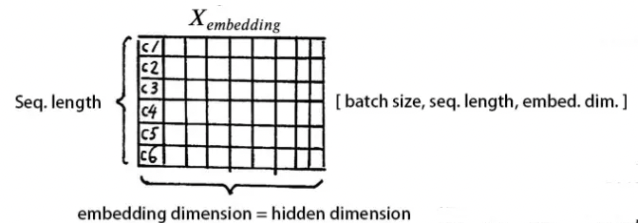Tranformer 采用的是 sin-cos 规则，使用了 sincos 函数的线性变换来提供给模型位置信息：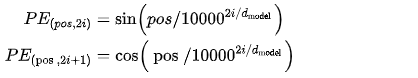# 导入依赖库
import numpy as np
import matplotlib.pyplot as plt
import seaborn as sns
import math

def get_positional_encoding(max_seq_len, embed_dim):
# 初始化一个positional encoding
# embed_dim: 字嵌入的维度
# max_seq_len: 最大的序列长度
positional_encoding = np.array([
[pos / np.power(10000, 2 * i / embed_dim) for i in range(embed_dim)]
if pos != 0 else np.zeros(embed_dim) for pos in range(max_seq_len)])
positional_encoding[1:, 0::2] = np.sin(positional_encoding[1:, 0::2])  # dim 2i 偶数
positional_encoding[1:, 1::2] = np.cos(positional_encoding[1:, 1::2])  # dim 2i+1 奇数
# 归一化, 用位置嵌入的每一行除以它的模长
# denominator = np.sqrt(np.sum(position_enc**2, axis=1, keepdims=True))
# position_enc = position_enc / (denominator + 1e-8)
return positional_encoding

positional_encoding = get_positional_encoding(max_seq_len=100, embed_dim=16)
plt.figure(figsize=(10,10))
sns.heatmap(positional_encoding)
plt.title("Sinusoidal Function")
plt.xlabel("hidden dimension")
plt.ylabel("sequence length")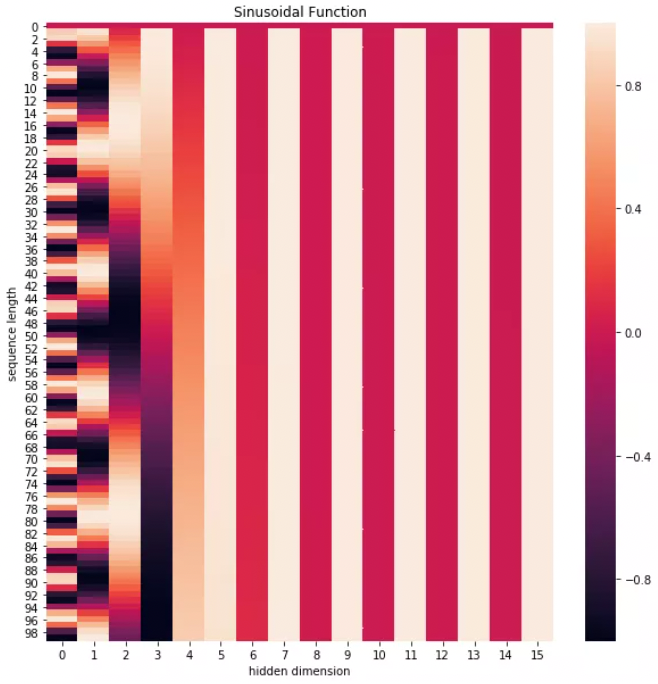## 2. 自注意力层（self-attention mechanism）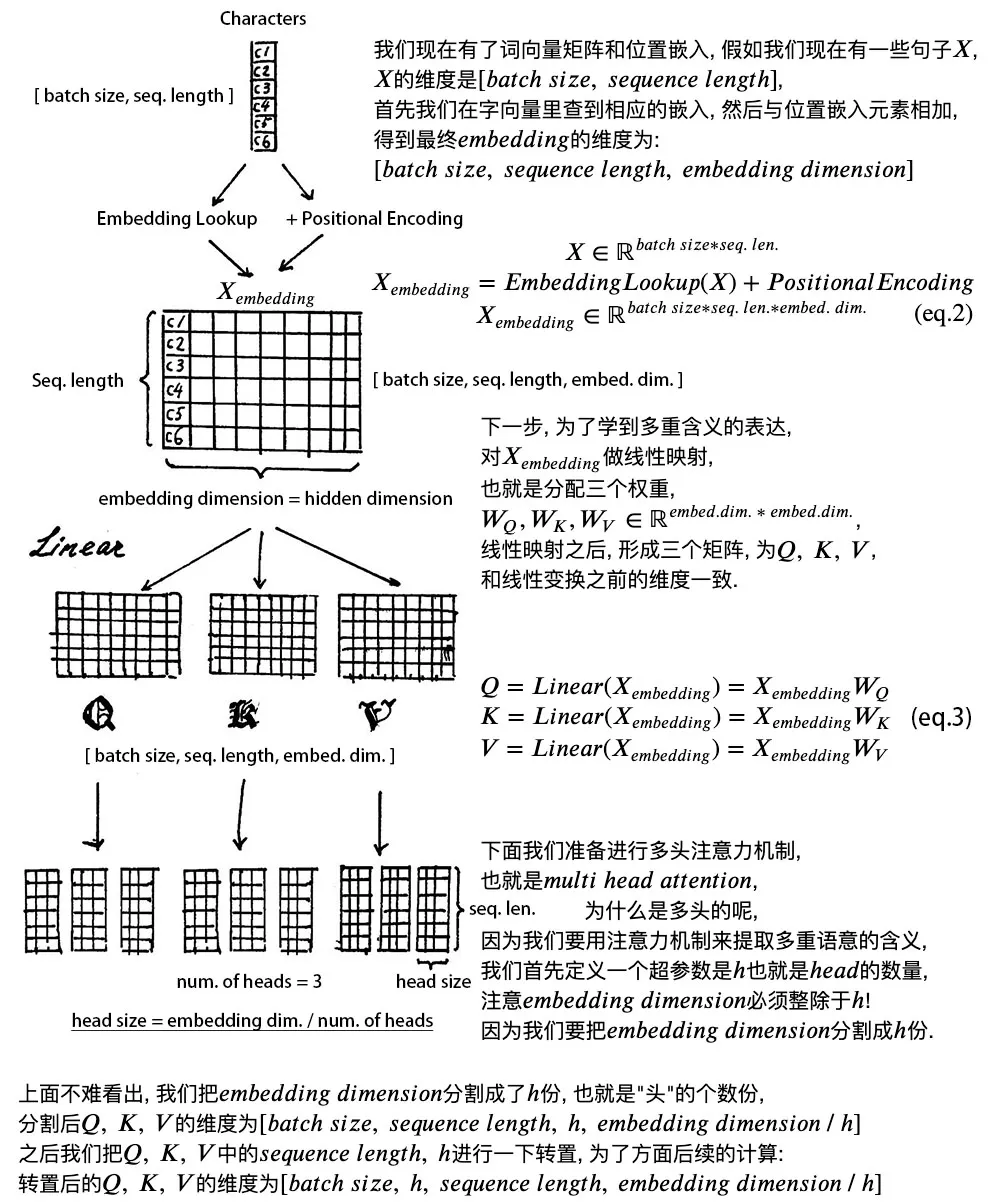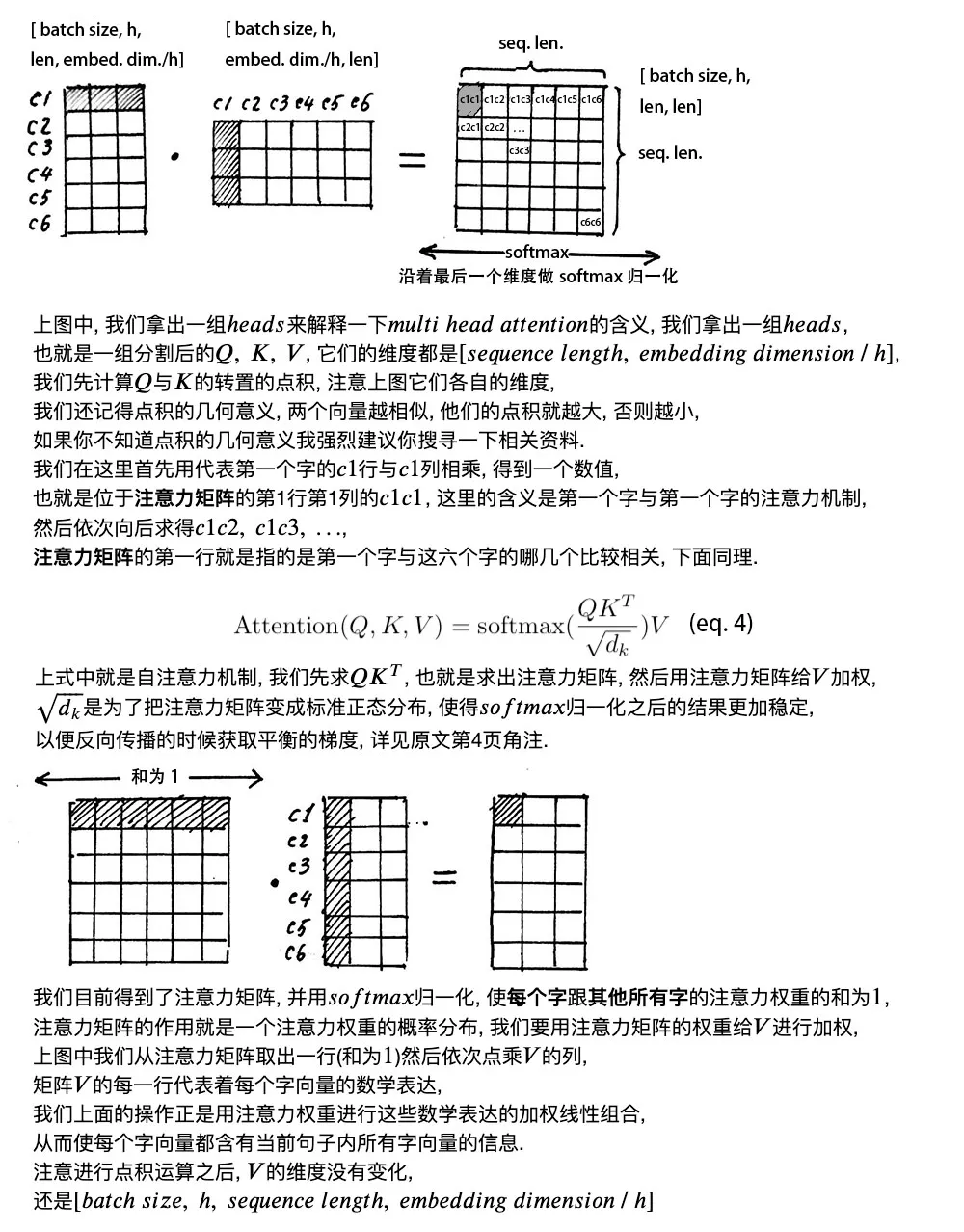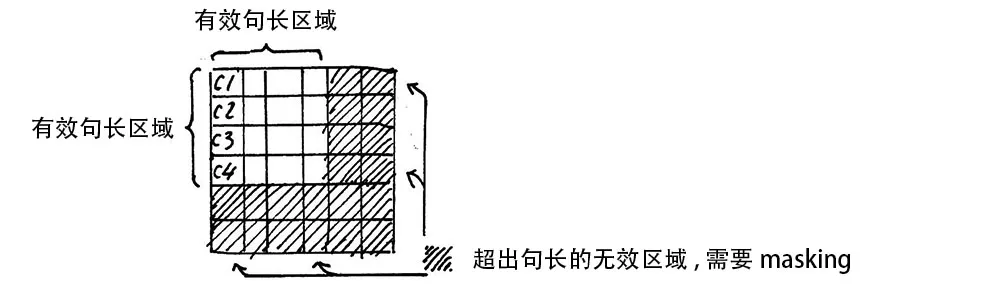## 3. 残差链接和层归一化

### 3.1. 残差设计

$$X_{embedding} + Attention(Q, K, V)$$

$$X + SubLayer(X)$$

### 3.2. 层归一化

$$\mu_i = \frac{1}{m} \sum_{i=1}^m x_{ij}$$

$$\sigma_{j}^2 = \frac{1}{m} \sum_{i=1}^m (x_{ij} - \mu_j)^2$$

$$LayerNorm(x) = \alpha \odot \frac{x_{ij} - \mu_i}{\sqrt{\sigma_i^2 + \epsilon}}$$

class ScaledDotProductAttention(nn.Module):
''' Scaled Dot-Product Attention '''
def __init__(self, temperature, attn_dropout=0.1):
super().__init__()
self.temperature = temperature
self.dropout = nn.Dropout(attn_dropout)

def forward(self, q, k, v, mask=None):
# self.temperature是论文中的d_k ** 0.5，防止梯度过大
# QxK/sqrt(dk)
attn = torch.matmul(q / self.temperature, k.transpose(2, 3))

# 屏蔽不想要的输出
# softmax+dropout
attn = self.dropout(F.softmax(attn, dim=-1))
# 概率分布xV
output = torch.matmul(attn, v)

return output, attn

Multi-Head Attention 实现在 ScaledDotProductAttention 基础上构建：

class MultiHeadAttention(nn.Module):

# d_model编码向量长度，例如本文说的512
# d_k, d_v的值一般会设置为 n_head * d_k=d_model，
# 此时concat后正好和原始输入一样，当然不相同也可以，因为后面有fc层
def __init__(self, n_head, d_model, d_k, d_v, dropout=0.1):
super().__init__()
self.d_k = d_k
self.d_v = d_v
# 可学习W^Q，W^K,W^V矩阵参数初始化
self.w_qs = nn.Linear(d_model, n_head * d_k, bias=False)
self.w_ks = nn.Linear(d_model, n_head * d_k, bias=False)
self.w_vs = nn.Linear(d_model, n_head * d_v, bias=False)
# 最后的输出维度变换操作
self.fc = nn.Linear(n_head * d_v, d_model, bias=False)
# 单头自注意力
self.attention = ScaledDotProductAttention(temperature=d_k ** 0.5)
self.dropout = nn.Dropout(dropout)
# 层归一化
self.layer_norm = nn.LayerNorm(d_model, eps=1e-6)

def forward(self, q, k, v, mask=None):
# 假设qkv输入是(b,100,512),100是训练每个样本最大单词个数
# 一般qkv相等，即自注意力
residual = q
# 将输入x和可学习矩阵相乘，得到(b,100,512)输出
# q的输出是(b,100,8,64),kv也是一样
q = self.w_qs(q).view(sz_b, len_q, n_head, d_k)
k = self.w_ks(k).view(sz_b, len_k, n_head, d_k)
v = self.w_vs(v).view(sz_b, len_v, n_head, d_v)

# 变成(b,8,100,64)，方便后面计算，也就是8个头单独计算
q, k, v = q.transpose(1, 2), k.transpose(1, 2), v.transpose(1, 2)

# 输出q是(b,8,100,64),维持不变,内部计算流程是：
# q*k转置，除以d_k ** 0.5，输出维度是b,8,100,100即单词和单词直接的相似性
# 对最后一个维度进行softmax操作得到b,8,100,100
# 最后乘上V，得到b,8,100,64输出

# b,100,8,64-->b,100,512
q = q.transpose(1, 2).contiguous().view(sz_b, len_q, -1)
q = self.dropout(self.fc(q))
# 残差计算
q += residual
# 层归一化，在512维度计算均值和方差，进行层归一化
q = self.layer_norm(q)

return q, attn

## 4. 前馈网络

class PositionwiseFeedForward(nn.Module):
''' A two-feed-forward-layer module '''
def __init__(self, d_in, d_hid, dropout=0.1):
super().__init__()
# 两个fc层，对最后的512维度进行变换
self.w_1 = nn.Linear(d_in, d_hid) # position-wise
self.w_2 = nn.Linear(d_hid, d_in) # position-wise
self.layer_norm = nn.LayerNorm(d_in, eps=1e-6)
self.dropout = nn.Dropout(dropout)

def forward(self, x):
residual = x

x = self.w_2(F.relu(self.w_1(x)))
x = self.dropout(x)
x += residual

x = self.layer_norm(x)

return x

## 5. Encoder 整体结构

 - 字向量与位置编码

$$X = EmbeddingLookup(X) + PositionalEncoding$$

$$X \in R^{batch\_size * seq\_len * embed\_dim}$$

 - 自注意力机制

$$Q = Linear(X) = XW_Q$$

$$K = Linear(X) = XW_K$$

$$V = Linear(X) = XW_V$$

$$X_{attention} = SelfAttention(Q, K, V)$$

 - 残差连接与层归一化

$$X_{attention} = X + X_{attenation}$$

$$X_{attention} = LayerNorm(X_{attention})$$

 - 前向网络

$$X_{hidden} = Activate(Linear(Linear(X_{attention})))$$

 - 重复

$$X_{hidden} = X_{attention} + X_{hidden}$$

$$X_{hidden} = LayerNorm(X_{hidden})$$

$$X_{hidden} \in R^{batch\_size * seq\_len * embed\_dim}$$

Last modification：August 25th, 2021 at 01:52 pm